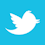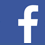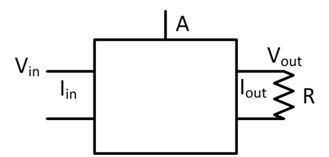By controlling the geometry, we can control reflections. But the rules of conduction are the same.

My recent article on current flow1 has sparked some interesting comments. And based on those comments, there is also still some confusion. I would like to highlight a couple of points and add a couple more.

First, the fundamental misunderstanding in talking about electrons as the flow of current is the velocity of the electrons. As I pointed out in my article, there are two velocities: the drift velocity of individual electrons, and the movement of energy through electrons so that we can have an electron exit a conductor after an electron enters the conductor, seemingly at the speed of light. We should forget about the drift velocity; it is not an issue in any way. When an electron (current) enters a conductor (probably driven by a voltage), it “collides” with another electron, which then collides with another electron, and so on. The interaction involves the transfer of energy (probably through photon interaction) among electrons. (This is not the same thing as a “wave.” This is a series of independent events.) This continues at the speed of light along the length of the conductor. The last electron in the chain is the one that leaves the conductor. This is how current (electrons) travels at the speed of light.

Some argue that when a signal travels along a transmission line, this is somehow different from a signal on a conductor. First, recall that current (signals) flows in a loop. (For good signal integrity, we strive to minimize this loop.) The only difference between a “conductor” and a transmission line is that a transmission line is a conductor with a controlled geometry. By controlling the geometry, we obtain some other benefits (such as controlling reflections). Otherwise, the rules of conduction are the same.

A second issue is what comes first, the current or the electromagnetic (EM) field? Consider a battery connected to a conductor through a switch. Switch the switch on and off as fast as you want. Now, what causes the current to flow in the conductor: the switched voltage (driving electrons into the conductor), or the EM field around the conductor? If it is the EM field, how did it get there? It is the switched current that happens first, with the EM field being a consequence of Ampere’s Law. The EM field does not induce the current; the current generates the EM field. Current comes first.

The third issue is how does radiation/transmission relate to all this? My initial article addressed the current flowing in a conductor only. Some occasionally confuse transmission with current flow. Consider the circuit in FIGURE 1.Figure 1. Circuit for illustration.

Assume whatever is in the box is a sealed/lossless circuit. Conductors probably are inside the box; they are probably carrying signal currents, and EM fields probably are around the conductors. The power into the box is Vin x Iin. The power out of the box is Vout x Iout. If everything is lossless, these two powers are equal. Now assume there is an antenna, A, out of the box. This antenna may be intentional (e.g., a radio, telephone, or Bluetooth antenna), or it may be unintentional (e.g., a source of EMI.) This antenna will radiate energy in the form of an electromagnetic wave. (Remember, energy is power x time.) In any event, the energy contained in the radiated field comes from, and is lost to, the circuit in the box. So the output power (Vout x Iout) will be reduced by the power in the radiated signal. In simple terms, the output current is reduced. Where did it go? It was absorbed in the radiation resistance.2

Now the radiated (transmitted) signal away from the box may contain one or more signals, as well as some noise. It does not contain any electrons. (By conservation of energy, they must remain in the conductor.) More important, the radiated energy, by itself, is pretty much useless – until the EM field interacts with a conductor somewhere.

If and when the radiated field intersects a conductor (e.g., receiving antenna), by Faraday’s Law, a current (flow of electrons) will be induced in that conductor. That current will generate a voltage. The product of that current and voltage represents power introduced into the conductor. That power is lost to the EM field (the opposite of what happened before).

Here is the important point. These two events (the transmission of the “signal” and the reception of the “signal”) are totally independent events. We can’t say they are part of the same circuit because they happen at different places and at different times. We may work hard to control those places and times for specific system purposes, but they are nonetheless independent events. The transmitting circuit does not know the receiving circuit is there, and the receiving circuit does not know the transmitting circuit is there. They each just “do their own thing.” In no way are the currents at the two locations related, as in being part of a single cohesive circuit.

So, back to our original circuit. The current is the movement of electrons. While any individual electron travels at a very slow velocity, the energy associated with an individual electron transfers between electrons (probably through photon interaction) at the speed of light. That is how electrons appear at the opposite end of the conductor so quickly. The movement of electrons creates an electromagnetic field around the conductor (not the other way around). This field travels in the form of a wave along the conductor, coupled to the electron energy. Some of the energy of the electromagnetic wave may be radiated away from the conductor; if so, that energy is lost to the circuit forever. We account for that loss by means of a virtual resistance we call “radiation resistance.”3 Whether or not that radiated energy is absorbed in another part of our system is a totally separate, independent discussion.

Notes

1. Douglas Brooks, Ph.D., “Is Electrical Current Particle Flow or a Wave Phenomenon?” PCD&F/CIRCUITS ASSEMBLY, February 2018.